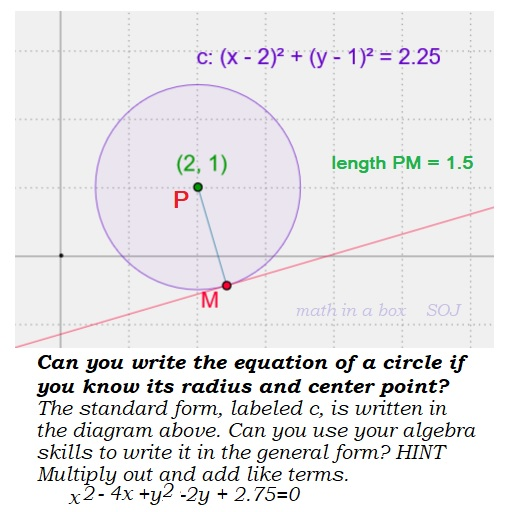# Circles in Algebra

Posted on: April 15, 2020 Posted by: Susan Johnsey Comments: 0The standard form of the equation of the circle is: (x – h)2 + (y – k)2 = r2    where the r is the radius and the point (h, k) is the center. If the center of the circle is the origin (0, 0), then the equation becomes x2 + y2 = r2. I am sure you know (x- 0)2 =x2 and (y- 0)2 =y2

Notice we have subtraction inside the parentheses, but addition between them!

If the center is point ( 2, -5) then that means h = 2 and k= -5.   Let 15 be the radius of the circle.  Our equation becomes

(x (2) )2 + (y (-5) )2 = 152 .  Simplify inside the parentheses first!

#### (x – 2)2 + (y +5)2 = 225.

Now let us use algebra to square each pair of parentheses.

#### Be careful!     (x – 2)2 is NOT x2 + 4.

To square these I suggest writing them twice and then multiply.    SEE: (x – 2 )(x – 2) = x2 -2x -2x +4 = x2 -4x+4. Now do the same for the (y +5)2   You will have y2 +10y +25.

Add together:  x2 – 4x+4 + y2 +10y +25 = 225.    And simplify.

x2 -4x + y2 +10y +29= 225  becomes

x2 -4x + y2 +10y -196=0 . This is the GENERAL form of the circle.